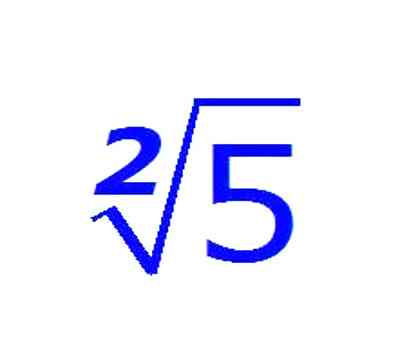Definitions of surds: A root of a positive real quantity is called a surd if its value cannot be exactly determined. It is a number that can’t be simplified to remove a square root (or cube root etc). For example, each of the quantities √3, ∛7, ∜19, (16)^2/5 etc. is a surd.

More Examples:

• √2 (square root of 2) can’t be simplified further so it is a surd
• √4 (square root of 4) CAN be simplified to 2, so it is NOT a surd

From the definition it is evident that a surd is an incommensurable quantity, although its value can be determined to any degree of accuracy. It should be noted that quantities √9, ∛64, ∜(256/625) etc. expressed in the form of surds  are commensurable quantities and are not surds (since √9 = 3, ∛64 = 4, ∜(256/625) = 4/5 etc.). In fact, any root of an algebraic expression is regarded as a surd.

Thus, each of √m, ∛n, 2√5×25 etc. may be regarded as a surd when the value of m (or n or x) is not given. Note that √m = 8 when m = 64; hence, in this case √m does not represent a surd. Thus, √m does not represent surd for all values of m.The surd symbol, all by itself, indicates the square root (1/2 power). If the surd has a number x written ahead of it and over the horizontal portion, then the symbol refers to the x th root (1/ x power) of that number. Therefore, for example:

3√ – indicates the cube root (1/3 power)

4√ – indicates the fourth root (1/4 power)

-7√ – indicates the -7th root (-1/7 power)

n√ – indicates the n th root (1/ n power)

The extent of the quantity affected by the surd, and that follows it, is indicated by placing a horizontal line over that quantity. The most common example is the square root symbol .

Note: All surds are irrationals but all irrational numbers are not surds. Irrational numbers like π and e, which are not the roots of algebraic expressions, are not surds.

Information Source: